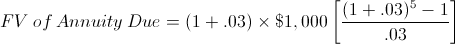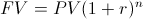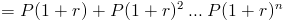# Future Value of Annuity DueThe future value of annuity due formula is used to calculate the ending value of a series of payments or cash flows where the first payment is received immediately. The first cash flow received immediately is what distinguishes an annuity due from an ordinary annuity. An annuity due is sometimes referred to as an immediate annuity.

The future value of annuity due formula calculates the value at a future date. The use of the future value of annuity due formula in real situations is different than that of the present value for an annuity due. For example, suppose that an individual or company wants to buy an annuity from someone and the first payment is received today. To calculate the price to pay for this particular situation would require use of the present value of annuity due formula. However, if an individual is wanting to calculate what their balance would be after saving for 5 years in an interest bearing account and they choose to put the first cash flow into the account today, the future value of annuity due would be used.

## Example of Future Value of Annuity Due Formula

To elaborate on the prior example of the future value of an annuity due, suppose that an individual would like to calculate their future balance after 5 years with today being the first deposit. The amount deposited per year is \$1,000 and the account has an effective rate of 3% per year. It is important to note that the last cash flow is received one year prior to the end of the 5th year.

For this example, we would use the future value of annuity due formula to come to the following equation:After solving, the balance after 5 years would be \$5468.41.

## How is the Future Value of Annuity Due Formula Derived?

There are a few different ways to determine the future value of annuity due formula. The first way is that we know thatThis means that we can multiply the present value of annuity due formula by (1+r)n. The present value of annuity due formula isNotice that if we multiply the 2nd portion of this formula by (1+r)n, the numerator becomes (1+r)n - 1, which is the same formula shown at the top of this page.

The second way to determine the future value of annuity due formula is to compare cash flows between an ordinary annuity and an annuity due. The cash flows for an annuity due arewhereas the cash flows for an ordinary annuity areOnce (1+r) is factored out of future value of annuity due cash flows, it becomes equal to the cash flows from an ordinary annuity. Therefore, the future value of an annuity due can be calculated by multiplying the future value of an ordinary annuity by (1+r), which is the formula shown at the top of the page.

New to Finance?